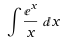# Computation Contest #5 Results and Solution## Solution

The problem of this contest was to solve the exponential integral numerically using taylor series from 0.9 to 1.1:First of all I want to apologize for telling you to solve a problem with a method that cannot be used to solve it, but learning by mistakes is always the best way to learn.

And now, here is my code:

``````public class TaylorIntegration {
// x!
static double faculty(int x) {
double res = 1;
for(int i = 2; i <= x; i++) {
res *= i;
}
return res;
}
// Generates the ferst terms of the taylor polynomial of e^x at x.
static double[] generateTaylorExp(int terms, double x) {
double[] coefficients = new double[terms];
double y = Math.exp(x);
for(int i = 0; i < terms; i++) {
coefficients[i] = y/faculty(i);
}
return coefficients;
}

// Generates the first terms of the taylor polynomial of a power function.
static double[] generateTaylorPower(double coef, int power, int terms, double x) {
double[] coefficients = new double[terms];
for(int i = 0; i < terms; i++) {
// Generate the coefficient:
coefficients[i] = coef*Math.pow(x, power)/faculty(i);
// Differentiate the function:
coef *= power;
power--;
}
return coefficients;
}

public static void main(String[] args) {
int size = Integer.parseInt(args); // Generate the taylor polynomial with 100 terms
double x = 1;
double[] taylorExp = generateTaylorExp(size+1, x); // Generate one additional term to compensate from the loss when divided by x.
double c1 = taylorExp; // Coefficient in front of the 1/x term after dividing by x.
double [] taylorx = generateTaylorPower(c1, -1, size, x); // Generate a polynomial to approximate 1/x at x = 1;

// Create the total taylor polynomial by summing up the parts:
double [] taylor = new double[size];
for(int i = 0; i < size; i++) {
taylor[i] = taylorx[i]+taylorExp[i+1]; // Remember that exp is divided by x, so the actual powers are 1 less then the index.
}

// Integrate:
double[] integrated = new double[size+1]; // By integration the number of terms is increased, because of "+C"
for(int i = 0; i < size; i++) {
// Perform integraion using the power rule:
int newPow = i+1;
double newCoef = taylor[i]/(newPow);
integrated[i+1] = newCoef;
}

// Caclulate the integral you want:
double x1 = 0.9, x2 = 1.1;
double res = 0;
for(int i = 0; i <= size; i++) {
res += (Math.pow(x2, i) - Math.pow(x1, i))*integrated[i];
}
System.out.println(res);
}
}``````

And that gives the following results depending on the number of terms in the taylor expansion:
`99 → 180.5206161521858`
`100 → -194.07526420957734`
`101 → 213.90044721848423`

As you can see, it's a mess…

#### Why is that?

The problem lies in the function 1/x.
At x = 1 it's coefficients are always ±1. So if one adds higher terms they won't be reduced by some n! like with e^x and so they will get really high for the upper border of the integral since 1.1^x grows to infinity. Because of the ±1 term the result changes between positive and negative.

Even when trying to integrate other points of this function the method it fails. Again because the derivatives of 1/x kill the 1/n! terms in the construction of the taylor series.

#### Is the whole method wrong then?

No!
The method is actually pretty good and most importantly it can be far more exact for small values of (x₂-x₁) than normal numerical integration.
The method is just not applicable for every function at every interval.

I hope you learned as much reading this as I did when programming this.

I think I will also make the next contest about this topic(Unless you protest).

↓↑↓↑↓↑↓↑↓↑↓↑↓↑↓↑↓↑↓↑↓↑↓↑↓↑↓↑↓↑↓↑↓↑↓↑↓↑↓↑↓↑↓↑↓↑↓↑↓

### List of participants with their entries:

Name Solution found Comment
@kaeserotor NONE, problems modelling taylor series I don't exactly know what problems you had, but maybe you discovered exactly what you where supposed to do, but then thought you did a mistake. Anyways because you are the only person who tried you will get the reward!

↓↑↓↑↓↑↓↑↓↑↓↑↓↑↓↑↓↑↓↑↓↑↓↑↓↑↓↑↓↑↓↑↓↑↓↑↓↑↓↑↓↑↓↑↓↑↓↑↓

## Winners:

Congratulations @kaeserotor, you won 2 SBI for trying to solve it!

↓↑↓↑↓↑↓↑↓↑↓↑↓↑↓↑↓↑↓↑↓↑↓↑↓↑↓↑↓↑↓↑↓↑↓↑↓↑↓↑↓↑↓↑↓↑↓↑↓

## Comments 1

Thanks a lot, I don't feel like I deserve any reward but thanks anyway :D
Also, I learned a lot trying this and even more through your explanation. Thank you!

22.11.2019 13:56
1